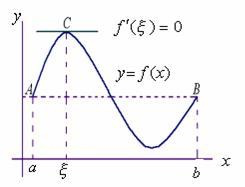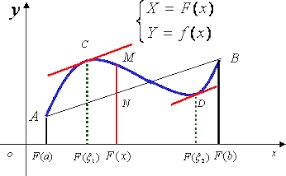# 高等数学——讲透微分中值定理

2019/04/10 10:10

<br>

<br>

## 费马引理

<br><br>

## 罗尔中值定理

<br>

1. 在闭区间[a, b]上连续
2. f(a) = f(b)
3. 在开区间(a, b)上可导

<br>

## 拉格朗日中值定理

<br>

1. 在闭区间[a, b]连续
2. 在开区间(a, b)可导

$$f(b) - f(a) = f'(\xi)(b - a)$$

$$f'(\xi) = \frac{f(b) - f(a)}{b - a}$$

$\frac{f(b) - f(a)}{b - a}$这个我们都非常熟悉，就是就是a和b两点连线的斜率。而$f'(\xi)$则是函数在$\xi$这点的切线，从几何角度上来看，说明存在一个点的切线和端点连线平行，我们可以对照下图。$$L(x) = f(x) - f(a) - \frac{f(b) - f(a)}{b - a}(x - a)$$

$$L'(x) = f'(x) - \frac{f(b) - f(a)}{b - a}$$

$$f'(\xi) - \frac{f(b)-f(a)}{b-a}=0$$

<br>

## 柯西中值定理

<br>1. 在闭区间[a, b]上连续
2. 在开区间(a, b)上可导
3. 对于任意$x \in (a, b), F'(x) \neq 0$

$$\frac{f(b) - f(a)}{F(b) - F(a)}=\frac{f'(\xi)}{F'(\xi)}$$

$$L(x) = f(x) - f(a)-\frac{f(b) - f(a)}{F(b) - F(a)}[F(x) - F(b)]$$0
0 收藏

### 作者的其它热门文章0 评论
0 收藏
0